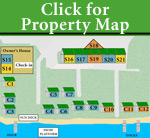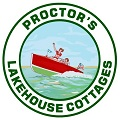Making Family Vacations
Special For Over 70 Years!
Lake Winnipesaukee, N.H.

< Back
You are here  >Availability                                    LAST UPDATED: August 17, 2019 "X" denotes already booked           "H" awaiting deposit          blank box denotes unit is available** All units are air-conditioned & heated and are NON-SMOKING inside, All units are self housekeeping (YOU DO) with cooking facilities. "C" units are Cottages  and    "S" units are Suites  (ALL UNITS HAVE A MAXIMUM NUMBER OF PERSONS- SEE UNIT SPECIFICS) **NOTE: Per night rates apply only to units not rented on a weekly basis.           Call us for 3 or 4 day COTTAGE availability & rental conditions-           2 WEEKS PRIOR TO DESIRED START DATE.

## Cottage & Suite Availability - July 2019

 Mon Tue Wed Thu Fri Sat Sun Mon Tue Wed Thu Fri Sat Sun Mon Tue Wed Thu Fri Sat Sun Mon Tue Wed Thu Fri Sat Sun Mon Tue Wed Unit Rooms/ Sleeps 1 2 3 4 5 6 7 8 9 10 11 12 13 14 15 16 17 18 19 20 21 22 23 24 25 26 27 28 29 30 31 Unit C-1 1/3 X X X X X X X X X X X X X X X X X X X X X X X X X C-1 C-2 2/6 X X X X X X X X X X X X X X X X X X X X X X X X X X X X X X C-2 C-3 3/6 X X X X X X X X X X X X X X X X X X X X X X X C-3 C-4 2/6 X X X X X X X X X X X X X X X X X X X X X X X X X X X X X X C-4 C-5 2/6 X X X X X X X X X X X X X X X X X X X X X X X X X X X X X C-5 C-6 2/6 X X X X X X X X X X X X X X X X X X X X X X X X X X X X X X C-6 C-7 2/6 X X X X X X X X X X X X X X X X X X X X X X X X X X X X X X X C-7 C-8 2/6 X X X X X X X X X X X X X X X X X X X X X X X X C-8 C-9 2/6 X X X X X X X X X X X X X X X X X X X X X X X X C-9 C-10 2/7 X X X X X X X X X X X X X X X X X X X X X X X X X X X X X X X C-10 C-11 2/7 X X X X X X X X X X X X X X X X X X X X X X X X X X X X X X X C-11 C-12 2/7 X X X X X X X X X X X X X X X X X X X X X X X X X X X C-12 S-14 1/3 X X X X X X X X X X X X X X X X X X X X X S-14 S-15 2/6 X X X X X X X X X X X X X X X S-15 S-16 1/3 X X X X X X X X X X X X X X X X X S-16 S-17 2/6 X X X X X X X X X X X X X X X X X X X X X X X S-17 S-18 2/6 X X X X X X X X X X X X X X X X X X X X X X X X X S-18 S-19 2/6 X X X X X X X X X X X X X X X X X X X X X X S-19 S-20 2/6 X X X X X X X X X X X X X X X X X X X X X X S-20 S-21 1/3 X X X X X X X X X X X X X X X X X X X X S-21 Mon Tue Wed Thu Fri Sat Sun Mon Tue Wed Thu Fri Sat Sun Mon Tue Wed Thu Fri Sat Sun Mon Tue Wed Thu Fri Sat Sun Mon Tue Wed 1 2 3 4 5 6 7 8 9 10 11 12 13 14 15 16 17 18 19 20 21 22 23 24 25 26 27 28 29 30 31Proctor's Lakehouse Cottages - NH
Waterfront Cottage Rentals , Suite Rentals & Condo Rentals on Lake Winnipesaukee, New Hampshire

(603) 366-5517 | info@lakehousecottages.com

GPS Coordinates: N43.36.056' W071.27.314'

Street Address: 1144 Weirs Boulevard, Laconia, NH 03246
Mailing Address: PO Box 5321, Weirs Beach, NH 03247-5321or text LAKEHOUSE to 22828.View our Photo Library